# GSEB Solutions Class 10 Maths Chapter 14 Statistics Ex 14.1

Gujarat Board GSEB Solutions Class 10 Maths Chapter 14 Statistics Ex 14.1 Textbook Questions and Answers.

## Gujarat Board Textbook Solutions Class 10 Maths Chapter 14 Statistics Ex 14.1

Question 1.
A survey was conducted by a group of students as a part of their environment awareness program, in which they collected the following data regarding the number of plants in 20 houses in a locality. Find the mean of plants per house.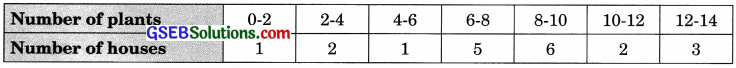Which method did you use for finding the mean and why?
Solution: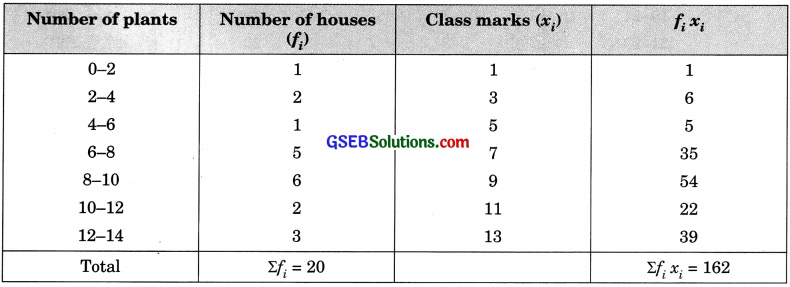$$\bar{x}$$ = $$\frac{\Sigma f_{i} x_{i}}{\Sigma f_{i}}$$ = $$\frac{162}{20}$$ = 8.1 plants
Direct method for finding the mean as numerical values of x. and f are small.

Question 2.
Consider the following distribution of daily wages of 50 workers of a factory.Find the mean daily wages of the workers of the factory by using an appropriate method.
Solution: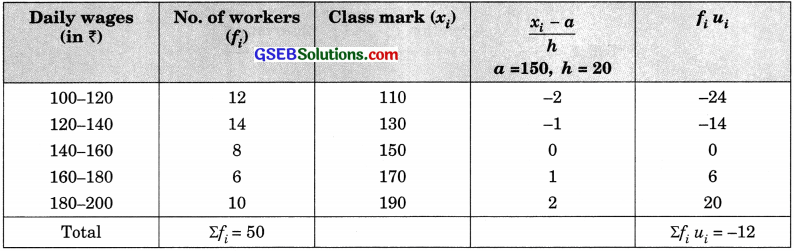Using the step-deviation method
$$\bar{x}$$ = a + $$\left(\frac{\Sigma f_{i} u_{i}}{\Sigma f_{i}}\right)$$ x h= 150 + $$\left(\frac{-12}{50}\right)$$ x 20 = 150 – 4.8 = 145.20
Hence, the mean daily wages of the workers of the factory is 145.20.Question 3.
The following distribution shows the daily pocket allowance of children of a locality. The mean pocket allowance is ₹ 18. Find the missing frequency f.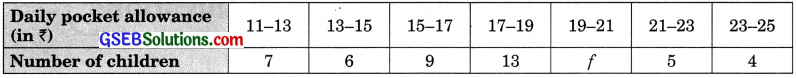Solution: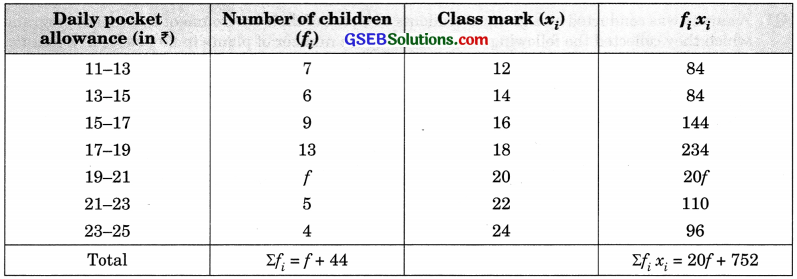Using the direct method,
$$\bar{x}$$ = $$\frac{\Sigma f_{i} x_{i}}{\Sigma f_{i}}$$
⇒ 18 = $$\frac{20 f+752}{f+44}$$
⇒ 20f + 752 = 18(f + 44)
⇒ 20f + 752 = 18f + 792
⇒ 20f – 18f = 792 – 752
2f = 40
f = $$\frac {4}{10}$$ = 20
Hence, the missing frequency is 20.

Question 4.
Thirty women were examined in a hospital by a doctor and the number of heartbeats per minute were recorded and summarized as follows. Find the mean heartbeat per minute for these women, choosing a suitable method.Solution:Using the step-deviation method
$$\bar{x}$$ = a + $$\left(\frac{\Sigma f_{i} u_{i}}{\Sigma f_{i}}\right)$$ x h = 75.5 + ($$\frac {4}{30}$$) x 3 = 75.5 + 0.4 = 75.9
Hence, the mean heart beat per minute is 75.9.Question 5.
In a retail market, fruit vendors were selling mangoes kept in packing boxes. These boxes contained varying numbers of mangoes. The following was the distribution of mangoes according to the number of boxes.Find the mean number of mangoes kept in a packing box.
Which method of finding the mean did you choose?
Solution: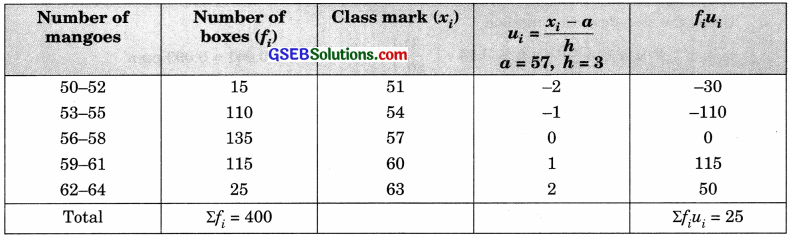Using the step-deviation method,
$$\bar{x}$$ = a + $$\left(\frac{\Sigma f_{i} u_{i}}{\Sigma f_{i}}\right)$$ x h = 57 + $$\left(\frac{25}{400}\right)$$ x 3 = 57 + 0.19 = 57.19

Question 6.
The table below shows the daily expenditure on the food of 25 households in a locality.Find the mean daily expenditure on food by a suitable method.
Solution: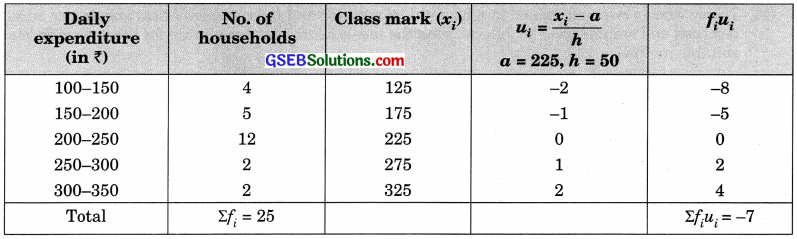Using the step-deviation method
$$\bar{x}$$ = a + $$\left(\frac{\Sigma f_{i} u_{i}}{\Sigma f_{i}}\right)$$ x h = 225 + ($$\frac {-7}{25}$$) x 50 = 225 – 14 = ₹ 211
Hence, the daily expeiiditure on food is 211.Question 7.
To find out the concentration of SO2 in the air (in parts per million, i.e. ppm), the data was collected for 30 localities in a certain n city and is presented below. Find the mean concentration of SO2 in the air.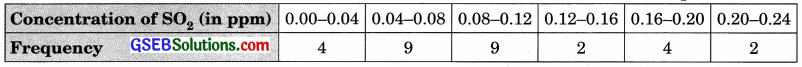Solution:Using the step-deviation method,
$$\bar{x}$$ = a + $$\left(\frac{\Sigma f_{i} u_{i}}{\Sigma f_{i}}\right)$$ x h = 0.14 + ($$\frac {-31}{30}$$) x 0.04 = 0.14 – 0.041 = 0.099 ppm
Therefore, the mean concentration of SO2 in the air is 0.099 ppm.

Question 8.
A class teacher has the following absent record of 40 students of a class for the whole term. Find the mean number of days a student was absent.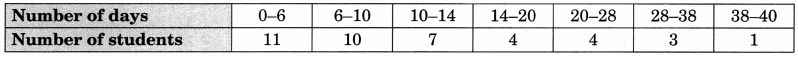Solution:Using direct method
$$\bar{x}$$ = $$\left(\frac{\Sigma f_{i} u_{i}}{\Sigma f_{i}}\right)$$ = $$\frac {499}{40}$$ = 12.47
Hence, the mean number of days a student was absent is 12.47.Question 9.
The following table gives the literacy rate (in percentage) of 35 cities. Find the mean literacy rate.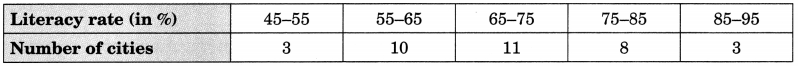Solution: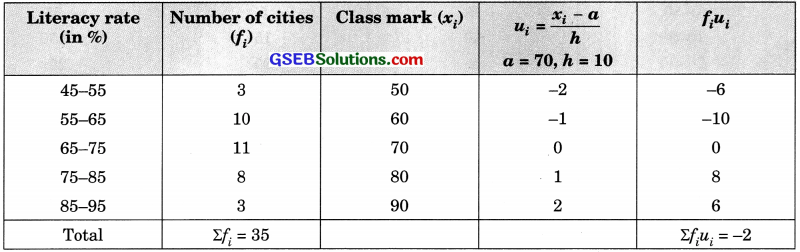Using the step-deviation method
$$\bar{x}$$ = a + $$\left(\frac{\Sigma f_{i} u_{i}}{\Sigma f_{i}}\right)$$ x h = 70 + $$\frac {-2}{35}$$ x 10 = 70 – $$\frac {4}{7}$$ = 70 – 0.57 = 69.43%
Hence, the literacy rate is 69.43%.